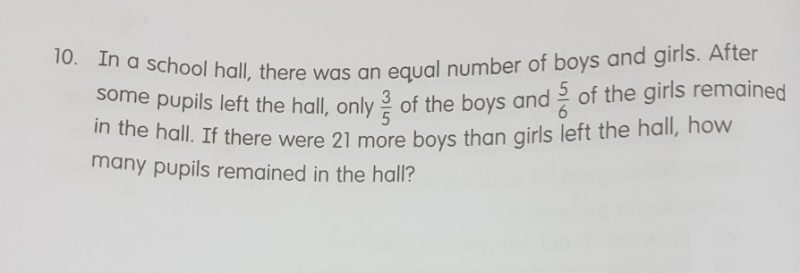# Question1 Answer

# Answer

Draw out the model : 1u = 1box

Equal number of boys & girls in hall:

Boys: 18u (remain) + 12u (left hall) = 30u

Girls: 25u (remain) + 5u (left hall) = 30u

12u – 5u = 21

7u = 21

u = 3

Number of pupils remaining in hall = 18u + 25u = 43u = 129

1 Reply 0 Likes

Thank you very much for the answer!😁

0 Replies 0 Likes